## Lewis Acids and Bases

### Learning Objectives

By the end of this module, you will be able to:

• Explain the Lewis model of acid-base chemistry
• Write equations for the formation of adducts and complex ions
• Perform equilibrium calculations involving formation constants

In 1923, G. N. Lewis proposed a generalized definition of acid-base behavior in which acids and bases are identified by their ability to accept or to donate a pair of electrons and form a coordinate covalent bond.

A coordinate covalent bond (or dative bond) occurs when one of the atoms in the bond provides both bonding electrons. For example, a coordinate covalent bond occurs when a water molecule combines with a hydrogen ion to form a hydronium ion. A coordinate covalent bond also results when an ammonia molecule combines with a hydrogen ion to form an ammonium ion. Both of these equations are shown here.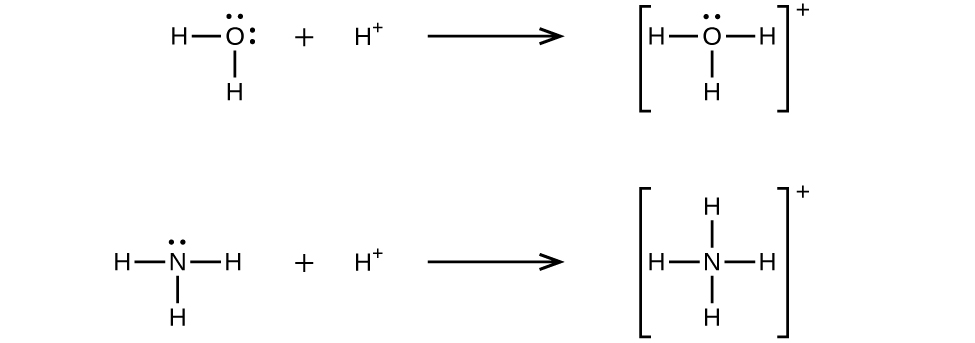A Lewis acid is any species (molecule or ion) that can accept a pair of electrons, and a Lewis base is any species (molecule or ion) that can donate a pair of electrons.

A Lewis acid-base reaction occurs when a base donates a pair of electrons to an acid. A Lewis acid-base adduct, a compound that contains a coordinate covalent bond between the Lewis acid and the Lewis base, is formed. The following equations illustrate the general application of the Lewis concept.

The boron atom in boron trifluoride, BF3, has only six electrons in its valence shell. Being short of the preferred octet, BF3 is a very good Lewis acid and reacts with many Lewis bases; a fluoride ion is the Lewis base in this reaction, donating one of its lone pairs: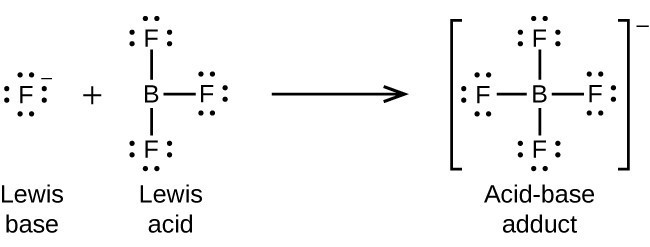In the following reaction, each of two ammonia molecules, Lewis bases, donates a pair of electrons to a silver ion, the Lewis acid: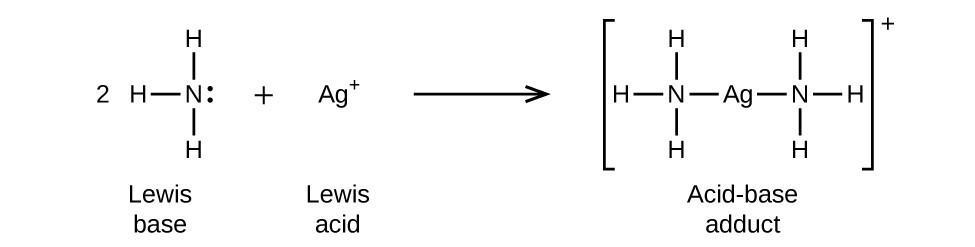Nonmetal oxides act as Lewis acids and react with oxide ions, Lewis bases, to form oxyanions: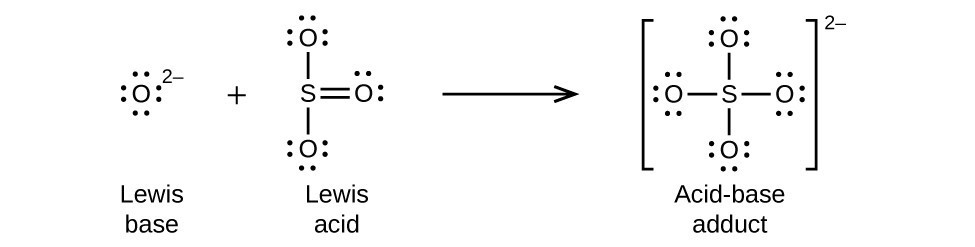Many Lewis acid-base reactions are displacement reactions in which one Lewis base displaces another Lewis base from an acid-base adduct, or in which one Lewis acid displaces another Lewis acid: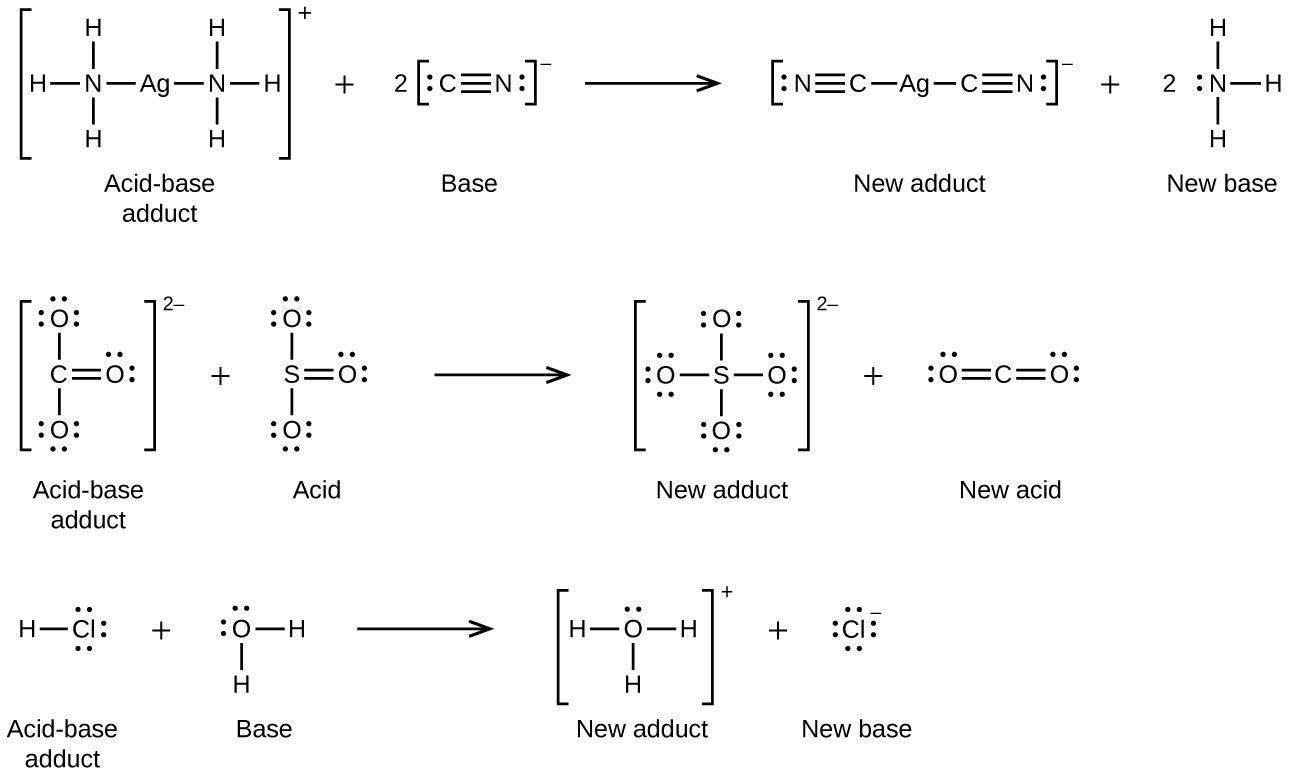The last displacement reaction shows how the reaction of a Brønsted-Lowry acid with a base fits into the Lewis concept. A Brønsted-Lowry acid such as HCl is an acid-base adduct according to the Lewis concept, and proton transfer occurs because a more stable acid-base adduct is formed. Thus, although the definitions of acids and bases in the two theories are quite different, the theories overlap considerably.

Many slightly soluble ionic solids dissolve when the concentration of the metal ion in solution is decreased through the formation of complex (polyatomic) ions in a Lewis acid-base reaction. For example, silver chloride dissolves in a solution of ammonia because the silver ion reacts with ammonia to form the complex ion $\text{Ag}{\left({\text{NH}}_{3}\right)}_{2}{}^{+}$. The Lewis structure of the $\text{Ag}{\left({\text{NH}}_{3}\right)}_{2}{}^{+}$ ion is: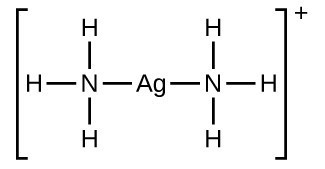The equations for the dissolution of AgCl in a solution of NH3 are:

$\begin{array}{rrll}{}&\text{AgCl}\left(s\right)&\longrightarrow&{\text{Ag}}^{\text{+}}\left(aq\right)+{\text{Cl}}^{-}\left(aq\right)\\{}&{\text{Ag}}^{\text{+}}\left(aq\right)+2{\text{NH}}_{3}\left(aq\right)&\longrightarrow&\text{Ag}{\left({\text{NH}}_{3}\right)}_{2}{}^{\text{+}}\left(aq\right)\\\text{Net: }&\text{AgCl}\left(s\right)+2{\text{NH}}_{3}\left(aq\right)&\longrightarrow &\text{Ag}{\left({\text{NH}}_{3}\right)}_{2}{}^{\text{+}}\left(aq\right)+{\text{Cl}}^{-}\left(aq\right)\end{array}$

Aluminum hydroxide dissolves in a solution of sodium hydroxide or another strong base because of the formation of the complex ion $\text{Al}{\left(\text{OH}\right)}_{4}{}^{-}$. The Lewis structure of the $\text{Al}{\left(\text{OH}\right)}_{4}{}^{-}$ ion is: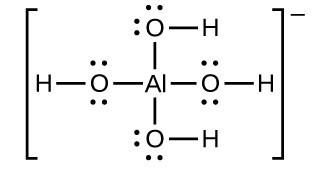The equations for the dissolution are:

$\begin{array}{rrll}{}&\text{Al}{\left(\text{OH}\right)}_{3}\left(s\right)&\longrightarrow& {\text{Al}}^{\text{3+}}\left(aq\right)+3{\text{OH}}^{-}\left(aq\right)\\{}&{\text{Al}}^{\text{3+}}\left(aq\right)+4{\text{OH}}^{-}\left(aq\right)&\longrightarrow&\text{Al}{\left(\text{OH}\right)}_{4}{}^{-}\left(aq\right)\\\text{Net:}&\text{Al}{\left(\text{OH}\right)}_{3}\left(s\right)+{\text{OH}}^{-}\left(aq\right)&\longrightarrow&\text{Al}{\left(\text{OH}\right)}_{4}{}^{-}\left(aq\right)\end{array}$

Mercury(II) sulfide dissolves in a solution of sodium sulfide because HgS reacts with the S2– ion:

$\begin{array}{rrll}{}&\text{HgS}\left(s\right)&\longrightarrow&{\text{Hg}}^{\text{2+}}\left(aq\right)+{\text{S}}^{2-}\left(aq\right)\\{}&{\text{Hg}}^{\text{2+}}\left(aq\right)+2{\text{S}}^{2-}\left(aq\right)&\longrightarrow&{\text{HgS}}_{2}{}^{2-}\left(aq\right)\\\text{Net:}&\text{HgS}\left(s\right)+{\text{S}}^{2-}\left(aq\right)&\longrightarrow &{\text{HgS}}_{2}{}^{2-}\left(aq\right)\end{array}$

A complex ion consists of a central atom, typically a transition metal cation, surrounded by ions, or molecules called ligands. These ligands can be neutral molecules like H2O or NH3, or ions such as CN or OH. Often, the ligands act as Lewis bases, donating a pair of electrons to the central atom. The ligands aggregate themselves around the central atom, creating a new ion with a charge equal to the sum of the charges and, most often, a transitional metal ion. This more complex arrangement is why the resulting ion is called a complex ion. The complex ion formed in these reactions cannot be predicted; it must be determined experimentally. The types of bonds formed in complex ions are called coordinate covalent bonds, as electrons from the ligands are being shared with the central atom. Because of this, complex ions are sometimes referred to as coordination complexes. This will be studied further in upcoming chapters.

The equilibrium constant for the reaction of the components of a complex ion to form the complex ion in solution is called a formation constant (Kf) (sometimes called a stability constant). For example, the complex ion $\text{Cu}{\left(\text{CN}\right)}_{2}{}^{-}$ is shown here: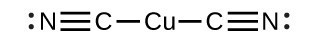It forms by the reaction:

${\text{Cu}}^{\text{+}}\left(aq\right)+2{\text{CN}}^{-}\left(aq\right)\rightleftharpoons \text{Cu}{\left(\text{CN}\right)}_{2}{}^{-}\left(aq\right)$

At equilibrium:

${K}_{\text{f}}=Q=\frac{\left[\text{Cu}{\left(\text{CN}\right)}_{2}{}^{-}\right]}{\left[{\text{Cu}}^{+}\right]{\left[{\text{CN}}^{-}\right]}^{2}}$

The inverse of the formation constant is the dissociation constant (Kd), the equilibrium constant for the decomposition of a complex ion into its components in solution. We will work with dissociation constants further in the exercises for this section. Formation Constants for Complex Ions and Table 1 are tables of formation constants. In general, the larger the formation constant, the more stable the complex; however, as in the case of Ksp values, the stoichiometry of the compound must be considered.

Table 1. Common Complex Ions by Decreasing Formulation Constants
Substance Kf at 25 °C
${\left[\text{Cd}{\left(\text{CN}\right)}_{4}\right]}^{2-}$ 1.3 $\times$ 107
$\text{Ag}{\left({\text{NH}}_{3}\right)}_{2}{}^{+}$ 1.7 $\times$ 107
${\left[{\text{AlF}}_{6}\right]}^{\text{3-}}$ 7 $\times$ 1019

As an example of dissolution by complex ion formation, let us consider what happens when we add aqueous ammonia to a mixture of silver chloride and water. Silver chloride dissolves slightly in water, giving a small concentration of Ag+ ([Ag+] = 1.3 $\times$ 10–5 M):

$\text{AgCl}\left(s\right)\rightleftharpoons {\text{Ag}}^{\text{+}}\left(aq\right)+{\text{Cl}}^{-}\left(aq\right)$

However, if NH3 is present in the water, the complex ion, $\text{Ag}{\left({\text{NH}}_{3}\right)}_{2}{}^{+}$, can form according to the equation:

${\text{Ag}}^{\text{+}}\left(aq\right)+2{\text{NH}}_{3}\left(aq\right)\rightleftharpoons \text{Ag}{\left({\text{NH}}_{3}\right)}_{2}{}^{\text{+}}\left(aq\right)$

with

${K}_{\text{f}}=\frac{\left[\text{Ag}{\left({\text{NH}}_{3}\right)}_{2}{}^{+}\right]}{\left[{\text{Ag}}^{+}\right]{\left[{\text{NH}}_{3}\right]}^{2}}=1.6\times {10}^{7}$

The large size of this formation constant indicates that most of the free silver ions produced by the dissolution of AgCl combine with NH3 to form $\text{Ag}{\left({\text{NH}}_{3}\right)}_{2}{}^{+}$. As a consequence, the concentration of silver ions, [Ag+], is reduced, and the reaction quotient for the dissolution of silver chloride, [Ag+][Cl], falls below the solubility product of AgCl:

$Q=\left[{\text{Ag}}^{+}\right]\left[{\text{Cl}}^{-}\right]<{K}_{\text{sp}}$

More silver chloride then dissolves. If the concentration of ammonia is great enough, all of the silver chloride dissolves.

### Example 1: Dissociation of a Complex Ion

Calculate the concentration of the silver ion in a solution that initially is 0.10 M with respect to $\text{Ag}{\left({\text{NH}}_{3}\right)}_{2}{}^{+}$.

Calculate the silver ion concentration, [Ag+], of a solution prepared by dissolving 1.00 g of AgNO3 and 10.0 g of KCN in sufficient water to make 1.00 L of solution. (Hint: Because Q < Kf, assume the reaction goes to completion then calculate the [Ag+] produced by dissociation of the complex.)

### Key Takeaways

G.N. Lewis proposed a definition for acids and bases that relies on an atom’s or molecule’s ability to accept or donate electron pairs. A Lewis acid is a species that can accept an electron pair, whereas a Lewis base has an electron pair available for donation to a Lewis acid. Complex ions are examples of Lewis acid-base adducts. In a complex ion, we have a central atom, often consisting of a transition metal cation, which acts as a Lewis acid, and several neutral molecules or ions surrounding them called ligands that act as Lewis bases. Complex ions form by sharing electron pairs to form coordinate covalent bonds. The equilibrium reaction that occurs when forming a complex ion has an equilibrium constant associated with it called a formation constant, Kf. This is often referred to as a stability constant, as it represents the stability of the complex ion. Formation of complex ions in solution can have a profound effect on the solubility of a transition metal compound.

### Exercises

1. Under what circumstances, if any, does a sample of solid AgCl completely dissolve in pure water?
2. Explain why the addition of NH3 or HNO3 to a saturated solution of Ag2CO3 in contact with solid Ag2CO3 increases the solubility of the solid.
3. Calculate the cadmium ion concentration, [Cd2+], in a solution prepared by mixing 0.100 L of 0.0100 M Cd(NO3)2 with 1.150 L of 0.100 NH3(aq).
4. Explain why addition of NH3 or HNO3 to a saturated solution of Cu(OH)2 in contact with solid Cu(OH)2 increases the solubility of the solid.
5. Sometimes equilibria for complex ions are described in terms of dissociation constants, Kd. For the complex ion ${\text{AlF}}_{6}{}^{\text{3-}}$ the dissociation reaction is:${\text{AlF}}_{6}^{3-}\rightleftharpoons {\text{Al}}^{\text{3+}}+6{\text{F}}^{-}$ and ${K}_{\text{d}}=\frac{\left[{\text{Al}}^{\text{3+}}\right]{\left[{\text{F}}^{-}\right]}^{6}}{\left[{\text{AlF}}_{6}^{3-}\right]}=2\times {10}^{-24}$Calculate the value of the formation constant, Kf, for ${\text{AlF}}_{\text{6}}^{\text{3}-}$.
6. Using the value of the formation constant for the complex ion $\text{Co}{\left({\text{NH}}_{3}\right)}_{6}{}^{\text{2+}}$, calculate the dissociation constant.
7. Using the dissociation constant, Kd = 7.8 $\times$ 10–18, calculate the equilibrium concentrations of Cd2+ and CN in a 0.250-M solution of $\text{Cd}{\left(\text{CN}\right)}_{4}^{2-}$.
8. Using the dissociation constant, Kd = 3.4 $\times$ 10–15, calculate the equilibrium concentrations of Zn2+ and OH in a 0.0465-M solution of $\text{Zn}{\left(\text{OH}\right)}_{4}^{2-}$.
9. Using the dissociation constant, Kd = 2.2 $\times$ 10–34, calculate the equilibrium concentrations of Co3+ and NH3 in a 0.500-M solution of $\text{Co}{\left({\text{NH}}_{3}\right)}_{6}^{\text{3+}}$.
10. Using the dissociation constant, Kd = 1 $\times$ 10–44, calculate the equilibrium concentrations of Fe3+ and CN in a 0.333 M solution of $\text{Fe}{\left(\text{CN}\right)}_{6}^{3-}$.
11. Calculate the mass of potassium cyanide ion that must be added to 100 mL of solution to dissolve 2.0 $\times$ 10–2 mol of silver cyanide, AgCN.
12. Calculate the minimum concentration of ammonia needed in 1.0 L of solution to dissolve 3.0 $\times$ 10–3 mol of silver bromide.
13. A roll of 35-mm black and white photographic film contains about 0.27 g of unexposed AgBr before developing. What mass of Na2S2O3.5H2O (sodium thiosulfate pentahydrate or hypo) in 1.0 L of developer is required to dissolve the AgBr as $\text{Ag}{\left({\text{S}}_{2}{\text{O}}_{3}\right)}_{2}{}^{\text{3-}}$ (Kf = 4.7 $\times$ 1013)?
14. We have seen an introductory definition of an acid: An acid is a compound that reacts with water and increases the amount of hydronium ion present. In the chapter on acids and bases, we saw two more definitions of acids: a compound that donates a proton (a hydrogen ion, H+) to another compound is called a Brønsted-Lowry acid, and a Lewis acid is any species that can accept a pair of electrons. Explain why the introductory definition is a macroscopic definition, while the Brønsted-Lowry definition and the Lewis definition are microscopic definitions.
15. Write the Lewis structures of the reactants and product of each of the following equations, and identify the Lewis acid and the Lewis base in each:
1. ${\text{CO}}_{2}+{\text{OH}}^{-}\longrightarrow {\text{HCO}}_{3}{}^{-}$
2. $\text{B}{\left(\text{OH}\right)}_{3}+{\text{OH}}^{-}\longrightarrow \text{B}{\left(\text{OH}\right)}_{4}{}^{-}$
3. ${\text{I}}^{-}+{\text{I}}_{2}\longrightarrow {\text{I}}_{3}{}^{-}$
4. ${\text{AlCl}}_{3}+{\text{Cl}}^{-}\longrightarrow {\text{AlCl}}_{4}{}^{-}$ (use Al-Cl single bonds)
5. ${\text{O}}^{2-}+{\text{SO}}_{3}\longrightarrow {\text{SO}}_{4}{}^{2-}$
16. Write the Lewis structures of the reactants and product of each of the following equations, and identify the Lewis acid and the Lewis base in each:
1. ${\text{CS}}_{2}+{\text{SH}}^{-}\longrightarrow {\text{HCS}}_{3}{}^{-}$
2. ${\text{BF}}_{3}+{\text{F}}^{-}\longrightarrow {\text{BF}}_{4}{}^{-}$
3. ${\text{I}}^{-}+{\text{SnI}}_{2}\longrightarrow {\text{SnI}}_{3}{}^{-}$
4. $\text{Al}{\left(\text{OH}\right)}_{3}+{\text{OH}}^{-}\longrightarrow \text{Al}{\left(\text{OH}\right)}_{4}{}^{-}$
5. ${\text{F}}^{-}+{\text{SO}}_{3}\longrightarrow {\text{SFO}}_{3}{}^{-}$
17. Using Lewis structures, write balanced equations for the following reactions:
1. $\text{HCl}\left(g\right)+{\text{PH}}_{3}\left(g\right)\longrightarrow$
2. ${\text{H}}_{3}{\text{O}}^{+}+{\text{CH}}_{3}{}^{-}\longrightarrow$
3. $\text{CaO}+{\text{SO}}_{3}\longrightarrow$
4. ${\text{NH}}_{4}{}^{+}+{\text{C}}_{2}{\text{H}}_{5}{\text{O}}^{-}\longrightarrow$
18. Calculate $\left[{\text{HgCl}}_{4}{}^{2-}\right]$ in a solution prepared by adding 0.0200 mol of NaCl to 0.250 L of a 0.100-M HgCl2 solution.
19. In a titration of cyanide ion, 28.72 mL of 0.0100 M AgNO3 is added before precipitation begins. [The reaction of Ag+ with CN goes to completion, producing the $\text{Ag}{\left(\text{CN}\right)}_{2}{}^{-}$ complex.] Precipitation of solid AgCN takes place when excess Ag+ is added to the solution, above the amount needed to complete the formation of $\text{Ag}{\left(\text{CN}\right)}_{2}{}^{-}$. How many grams of NaCN were in the original sample?
20. What are the concentrations of Ag+, CN, and $\text{Ag}{\left(\text{CN}\right)}_{2}{}^{-}$ in a saturated solution of AgCN?
21. In dilute aqueous solution HF acts as a weak acid. However, pure liquid HF (boiling point = 19.5 °C) is a strong acid. In liquid HF, HNO3 acts like a base and accepts protons. The acidity of liquid HF can be increased by adding one of several inorganic fluorides that are Lewis acids and accept F ion (for example, BF3 or SbF5). Write balanced chemical equations for the reaction of pure HNO3 with pure HF and of pure HF with BF3. Write the Lewis structures of the reactants and products, and identify the conjugate acid-base pairs.
22. The simplest amino acid is glycine, H2NCH2CO2H. The common feature of amino acids is that they contain the functional groups: an amine group, –NH2, and a carboxylic acid group, –CO2H. An amino acid can function as either an acid or a base. For glycine, the acid strength of the carboxyl group is about the same as that of acetic acid, CH3CO2H, and the base strength of the amino group is slightly greater than that of ammonia, NH3.
1. Write the Lewis structures of the ions that form when glycine is dissolved in 1 M HCl and in 1 M KOH.
2. Write the Lewis structure of glycine when this amino acid is dissolved in water. (Hint: Consider the relative base strengths of the –NH2 and $-{\text{CO}}_{2}{}^{-}$ groups.)
23. Boric acid, H3BO3, is not a Brønsted-Lowry acid but a Lewis acid.
1. Write an equation for its reaction with water.
2. Predict the shape of the anion thus formed.
3. What is the hybridization on the boron consistent with the shape you have predicted?

## Glossary

complex ion: ion consisting of a transition metal central atom and surrounding molecules or ions called ligands

dissociation constant: (Kd) equilibrium constant for the decomposition of a complex ion into its components in solution

formation constant: (Kf) (also, stability constant) equilibrium constant for the formation of a complex ion from its components in solution

Lewis acid: any species that can accept a pair of electrons and form a coordinate covalent bond

Lewis acid-base adduct: compound or ion that contains a coordinate covalent bond between a Lewis acid and a Lewis base

Lewis base: any species that can donate a pair of electrons and form a coordinate covalent bond

ligand: molecule or ion that surrounds a transition metal and forms a complex ion; ligands act as Lewis bases